Overview: LOGISTIC Procedure

Binary responses (for example, success and failure), ordinal responses (for example, normal, mild, and severe), and nominal responses (for example, major TV networks viewed at a certain hour) arise in many fields of study. Logistic regression analysis is often used to investigate the relationship between these discrete responses and a set of explanatory variables. Texts that discuss logistic regression include Agresti (2002), Allison (1999), Collett (2003), Cox and Snell (1989), Hosmer and Lemeshow (2000), and Stokes, Davis, and Koch (2000).

For binary response models, the response,, of an individual or an experimental unit can take on one of two possible values, denoted for convenience by 1 and 2 (for example,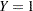if a disease is present, otherwise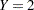). Supposeis a vector of explanatory variables and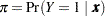is the response probability to be modeled. The linear logistic model has the form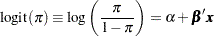whereis the intercept parameter and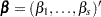is the vector ofslope parameters. Notice that the LOGISTIC procedure, by default, models the probability of the lower response levels.

The logistic model shares a common feature with a more general class of linear models: a function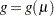of the mean of the response variable is assumed to be linearly related to the explanatory variables. Since the meanimplicitly depends on the stochastic behavior of the response, and the explanatory variables are assumed to be fixed, the functionprovides the link between the random (stochastic) component and the systematic (deterministic) component of the response variable. For this reason, Nelder and Wedderburn (1972) refer to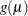as a link function. One advantage of the logit function over other link functions is that differences on the logistic scale are interpretable regardless of whether the data are sampled prospectively or retrospectively (McCullagh and Nelder; 1989, Chapter 4). Other link functions that are widely used in practice are the probit function and the complementary log-log function. The LOGISTIC procedure enables you to choose one of these link functions, resulting in fitting a broader class of binary response models of the form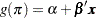For ordinal response models, the response,, of an individual or an experimental unit might be restricted to one of a (usually small) number of ordinal values, denoted for convenience by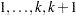. For example, the severity of coronary disease can be classified into three response categories as 1=no disease, 2=angina pectoris, and 3=myocardial infarction. The LOGISTIC procedure fits a common slopes cumulative model, which is a parallel lines regression model based on the cumulative probabilities of the response categories rather than on their individual probabilities. The cumulative model has the form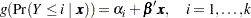whereareintercept parameters, andis the vector of slope parameters. This model has been considered by many researchers. Aitchison and Silvey (1957) and Ashford (1959) employ a probit scale and provide a maximum likelihood analysis; Walker and Duncan (1967) and Cox and Snell (1989) discuss the use of the log odds scale. For the log odds scale, the cumulative logit model is often referred to as the proportional odds model.

For nominal response logistic models, where the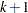possible responses have no natural ordering, the logit model can also be extended to a multinomial model known as a generalized or baseline-category logit model, which has the form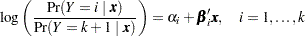where theareintercept parameters, and the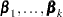arevectors of slope parameters. These models are a special case of the discrete choice or conditional logit models introduced by McFadden (1974).

The LOGISTIC procedure fits linear logistic regression models for discrete response data by the method of maximum likelihood. It can also perform conditional logistic regression for binary response data and exact logistic regression for binary and nominal response data. The maximum likelihood estimation is carried out with either the Fisher scoring algorithm or the Newton-Raphson algorithm, and you can perform the bias-reducing penalized likelihood optimization as discussed by Firth (1993) and Heinze and Schemper (2002). You can specify starting values for the parameter estimates. The logit link function in the logistic regression models can be replaced by the probit function, the complementary log-log function, or the generalized logit function.

Any term specified in the model is referred to as an effect. The LOGISTIC procedure enables you to specify categorical variables (also known as classification or CLASS variables) and continuous variables as explanatory effects. You can also specify more complex model terms such as interactions and nested terms in the same way as in the GLM procedure. You can create complex constructed effects with the EFFECT statement. An effect in the model that is not an interaction or a nested term or a constructed effect is referred to as a main effect.

The LOGISTIC procedure allows either a full-rank parameterization or a less-than-full-rank parameterization of the CLASS variables. The full-rank parameterization offers eight coding methods: effect, reference, ordinal, polynomial, and orthogonalizations of these. The effect coding is the same method that is used in the CATMOD procedure. The less-than-full-rank parameterization, often called dummy coding, is the same coding as that used in the GLM procedure.

The LOGISTIC procedure provides four effect selection methods: forward selection, backward elimination, stepwise selection, and best subset selection. The best subset selection is based on the likelihood score statistic. This method identifies a specified number of best models containing one, two, three effects, and so on, up to a single model containing effects for all the explanatory variables.

The LOGISTIC procedure has some additional options to control how to move effects in and out of a model with the forward selection, backward elimination, or stepwise selection model-building strategies. When there are no interaction terms, a main effect can enter or leave a model in a single step based on the p-value of the score or Wald statistic. When there are interaction terms, the selection process also depends on whether you want to preserve model hierarchy. These additional options enable you to specify whether model hierarchy is to be preserved, how model hierarchy is applied, and whether a single effect or multiple effects can be moved in a single step.

Odds ratio estimates are displayed along with parameter estimates. You can also specify the change in the continuous explanatory main effects for which odds ratio estimates are desired. Confidence intervals for the regression parameters and odds ratios can be computed based either on the profile-likelihood function or on the asymptotic normality of the parameter estimators. You can also produce odds ratios for effects that are involved in interactions or nestings, and for any type of parameterization of the CLASS variables.

Various methods to correct for overdispersion are provided, including Williams’ method for grouped binary response data. The adequacy of the fitted model can be evaluated by various goodness-of-fit tests, including the Hosmer-Lemeshow test for binary response data.

Like many procedures in SAS/STAT software that enable the specification of CLASS variables, the LOGISTIC procedure provides a CONTRAST statement for specifying customized hypothesis tests concerning the model parameters. The CONTRAST statement also provides estimation of individual rows of contrasts, which is particularly useful for obtaining odds ratio estimates for various levels of the CLASS variables. The LOGISTIC procedure also provides testing capability through the ESTIMATE and TEST statements. Analyses of LS-means are enabled with the LSMEANS, LSMESTIMATE, and SLICE statements.

You can perform a conditional logistic regression on binary response data by specifying the STRATA statement. This enables you to perform matched-set and case-control analyses. The number of events and nonevents can vary across the strata. Many of the features available with the unconditional analysis are also available with a conditional analysis.

The LOGISTIC procedure enables you to perform exact logistic regression, also known as exact conditional logistic regression, by specifying one or more EXACT statements. You can test individual parameters or conduct a joint test for several parameters. The procedure computes two exact tests: the exact conditional score test and the exact conditional probability test. You can request exact estimation of specific parameters and corresponding odds ratios where appropriate. Point estimates, standard errors, and confidence intervals are provided. You can perform stratified exact logistic regression by specifying the STRATA statement.

Further features of the LOGISTIC procedure enable you to do the following:

• control the ordering of the response categories

• compute a generalizedmeasure for the fitted model

• reclassify binary response observations according to their predicted response probabilities

• test linear hypotheses about the regression parameters

• create a data set for producing a receiver operating characteristic curve for each fitted model

• specify contrasts to compare several receiver operating characteristic curves

• create a data set containing the estimated response probabilities, residuals, and influence diagnostics

• score a data set by using a previously fitted model

The LOGISTIC procedure uses ODS Graphics to create graphs as part of its output. For general information about ODS Graphics, see Chapter 21, Statistical Graphics Using ODS. For more information about the plots implemented in PROC LOGISTIC, see the section ODS Graphics.

The remaining sections of this chapter describe how to use PROC LOGISTIC and discuss the underlying statistical methodology. The section Getting Started: LOGISTIC Procedure introduces PROC LOGISTIC with an example for binary response data. The section Syntax: LOGISTIC Procedure describes the syntax of the procedure. The section Details: LOGISTIC Procedure summarizes the statistical technique employed by PROC LOGISTIC. The section Examples: LOGISTIC Procedure illustrates the use of the LOGISTIC procedure.

For more examples and discussion on the use of PROC LOGISTIC, see Stokes, Davis, and Koch (2000), Allison (1999), and SAS Institute Inc. (1995).